# How to sum in excel(9 formulas), with shortcut keys and to a column or multiple rows

Lionsure 2020-08-06 Original by the website

In Excel, sum the table is one of the normal operations, it is used much more. There are two main methods for Excel sum. One is to use the sum option provided by Excel, and the other is to use shortcut keys. In terms of convenience and speed, it is convenient and fast to use the sum shortcut keys rather than the sum option.

You can sum a row or a column, or can also sum the specified ranges of  cell at a time; and it can sum multiple rows, columns, or multiple ranges of  cell. In addition, there is a shortcut method of sum for multi-row or multi-column in batches, that is, simply select the cell corresponding to the row or column required to achieve the summation.

### I, How to sum in excel method 1: Use the sum option

(1) How to sum a row in excel

1. If you want to count the total score of each student. Select cell E2, select the "Formulas" tab, click the "AutoSum" icon (i.e. ∑ icon) at the top left of the screen, then the sum formula =SUM(B2:D2) is filled automatically into the cell E2, press Enter on your keyboard, find the total score of the first student; move the mouse to the cell fill handle on the lower right corner of E2, hold down the left button of mouse, drag down until the last row, then count the total of all students. The process steps are as shown in Figure 1: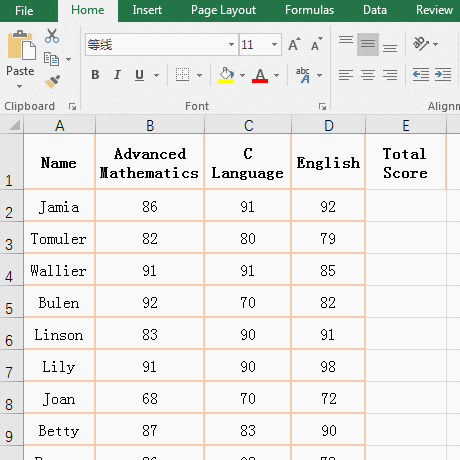Flgure 1

2. Tip: If you drag down the process, the cells that have passed do not become the total score of the corresponding student. After dragging to the last line, press Ctrl + S to save, it will be updated to the total score of the corresponding student.

(2) How to sum a column in excel

1. If you want to subtotal the sum of clothing sales. Select cell D11, select the "Formulas" tab, click the "AutoSum" icon (i.e. ∑ icon), then the sum formula =SUM(D2:D10) is filled automatically into D11, press Enter on the keyboard, and sum of the clothing sales  is subtotalled. the operating process steps, as shown in Figure 2: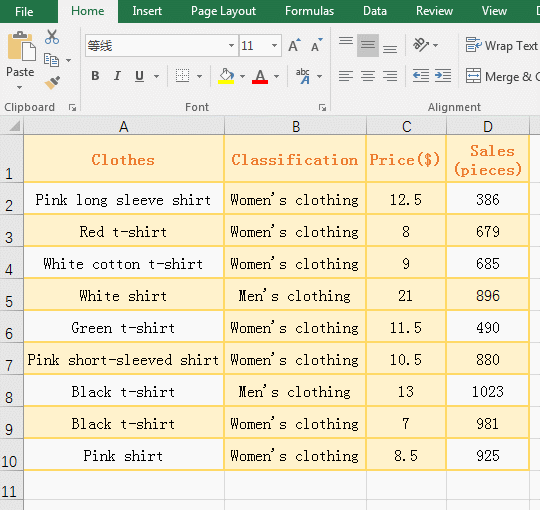Flgure 2

2. Tip: If you want to sum multiple columns, you can also use the drag method to find the sum of other columns after you subtotal a column. Note that you can only drag to the right, so you must first subtotal the sum of the leftmost column.

### II, How to sum in excel method 2: Quickly sum with shortcut keys

(1) How to sum a row in excel use shortcut keys

Also count the subtotal score of each student. Select cell E2, and press the shortcut keys Alt + =, then the sum formula =SUM(B2:D2) is filled automatically into the cell E2, press Enter to count the total score of the first student; move the mouse to the cell fill handle on the lower right corner of E2, after the mouse becomes the bold black plus sign(+), double-click the left button to count automatically the total score of each remaining student in the table; the operation steps are as shown in Figure 3: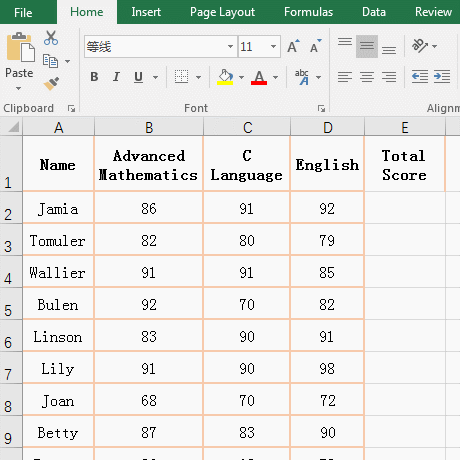Flgure 3

(2) How to sum a column in excel use shortcut keys

If you want to count the total score of each subject. Select cell B22, and press the shortcut keys Alt + =, then the sum formula =SUM(B2:B21) is filled automatically into the cell B22, press Enter, and the total score of the "Advanced Mathematics" is subtotalled; move the mouse to the cell fill handle on the lower right corner of B22, after the mouse becomes the bold black plus sign(+), hold down the left button, drag to the right until the last column, then count the total score of all subjects; the operation process steps, as shown in Figure 4: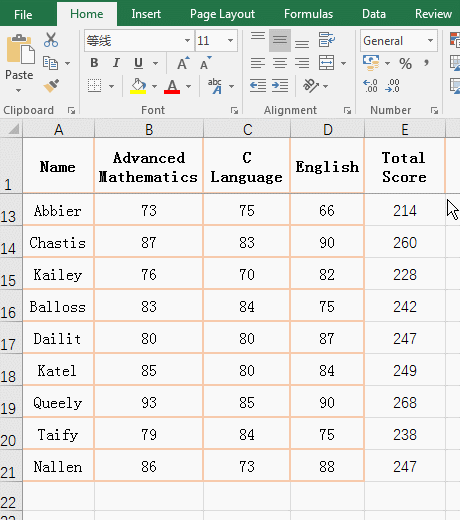Flgure 4

Hint: Sum of columns can't be like summing rows, you cann't find the sum of other columns by clicking the fill handle of the cell, so you can only drag to the right.

### III, How to sum in excel for multiple cells

(1) Method 1: Shortcut keys + frame selection

If you want the sum of clothing sales from January to March. Select cell F2, press the shortcut keys Alt + =, then enter automatically the formula =SUM(B2:E2), select B2:E4, then B2:E2 in the formula will change to B2:E4, press Enter, return to the sum of selected cells. the operation process steps, as shown in Figure 5: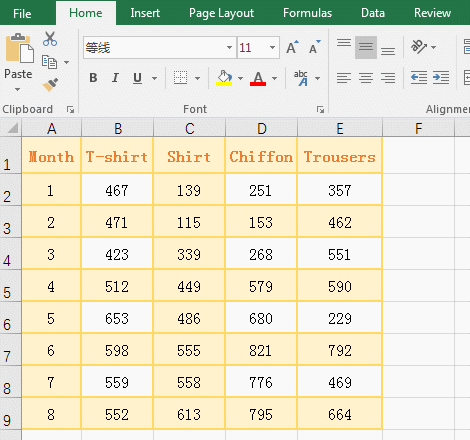Flgure 5

(2) Method 2: Input + frame selection

The same is true for the sum of apparel sales from January to March. Select the cell F2, enter the formula =sum(, select B2:E4, then enter the right parenthesis, press Enter, then find the sum of the clothing sales in the selected range. the operation process steps, as shown in Figure 6: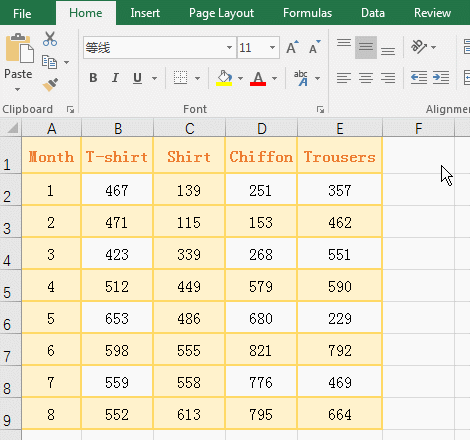Flgure 6

(3) Simultaneous sum of discontinuous cell ranges

Select the cell F2, enter the formula =sum(, select B2:C3, then enter the comma(,), select C5:D8, then enter the right parenthesis, press Enter, then find sum of the two selected cell ranges. The process steps, as shown in Figure 7: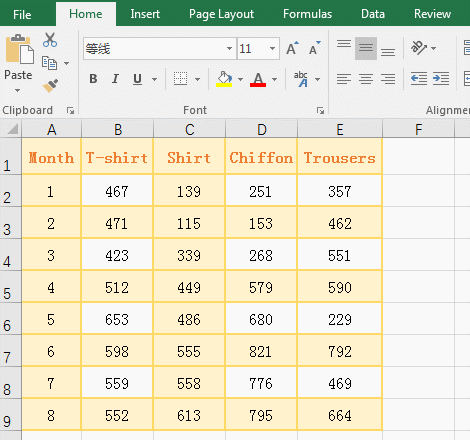Flgure 7

### IV, How to sum in excel for multiple rows or columns

(1) How to Sum columns in excel

1. If you want to sum the sales of all kinds of clothing in the clothing table. Select B2:E9, press the shortcut keys Alt + =, then find automatically the sum of all kinds of clothing sales. the operation process steps, as shown in Figure 8: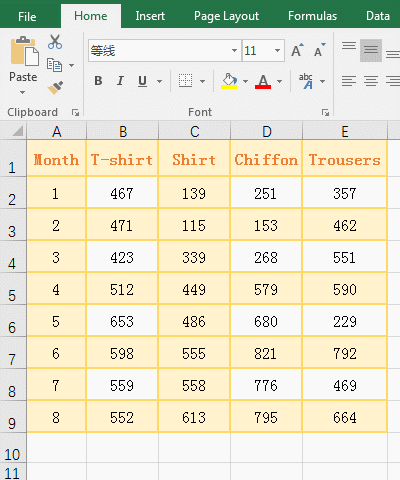Flgure 8

2. Tip: This method of sum may be convert automatically format of the cells that store the sum result into text. Double-click these cells again, and press Enter to not return automatically the sum result. You need to set the cell format to general. In addition, if the number of rows is particularly large and difficult to select, you can select the columns.

(2) How to sum rows in excel

If you want to sum the clothing sales of every month. Select F2:F9, press the shortcut keys Alt + =, then find automatically the sum of clothing sales from January to August. the operation process steps, as shown in Figure 9: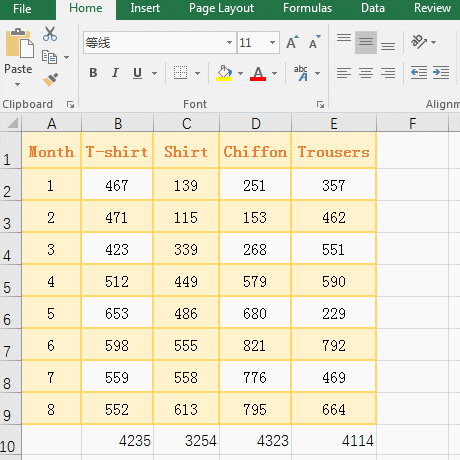Flgure 9

In addition to the above operations, you can also manually enter excel sum formula. For example, the sales of T-shirts are summed. Select cell B9, enter the formula =sum(b2:b9), press Enter, return to the result of sum ; the operation steps are as shown in Figure 10: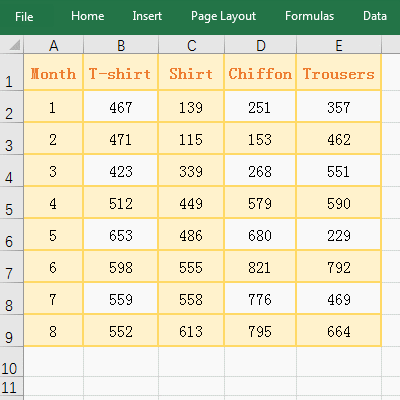Flgure 10